### Quant practice questions, sample math questionbank - Ascent Education XAT 2016 / TANCET 2018 classes, correspondence course

 Home TANCET Classes GMAT Coaching Online GMAT Course CAT Classes TANCET Study Material
 TANCET '18 Classroom Program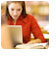Weekend and weekday classes for TANCET MBA @ Chennai. At Nungambakkam and Velachery.Other Courses TANCET Correspondence Course CAT Classes & Courses GMAT Classes Chennai Online GMAT Course GRE Classes Chennai CBSE Math Online Tuition SAT Classes Other Links Ascent TANCET Toppers Testimonials CAT, TANCET Questions Careers @ Ascent Contact Us +91 44 4500 8484 +91 96000 48484 ascent@ascenteducation.com Postal Address Facebook / Twitter / Blog / Videos
You are here: Home  »  XAT, TANCET Prep Questions »  Percents, Fractions »   Question 10

# Percentages : XAT 2016 / XAT 2016 Math Practice Question

This question is an arithmetic question from the topic Percentages.

## Question

Peter got 30% of the maximum marks in an examination and failed by 10 marks. However, Paul who took the same examination got 40% of the total marks and got 15 marks more than the passing marks. What was the passing marks in the examination?
1. 35
2. 250
3. 75
4. 85

85. Choice (4)

Let 'x' be the maximum marks in the examination.

Therefore, Peter got 30% of x =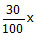= 0.3x
And Paul got 40% of x =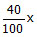= 0.4x.
In terms of the maximum marks Paul got 0.4x - 0.3x = 0.1x more than Peter. -- (1)
The problem however, states that Paul got 15 marks more than the passing mark and Peter got 10 marks less than the passing mark. Therefore, Paul has got 15 + 10 = 25 marks more than Peter. -- (2)

Equating (1) and (2), we get
0.1x = 25 => x =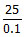= 250
'x' is the maximum mark and is equal to 250 marks.

We know that Peter got 30% of the maximum marks.

Therefore, Peter got= 75 marks.

We also know that Peter got 10 marks less than the passing mark.

Therefore, the passing mark will be 10 marks more than what Peter got = 75 + 10 = 85.

## CAT, XAT, TANCET Practice Questions and Answers : Listed Topicwise

 Number Theory Permutation Combination Probability Inequalities Geometry Mensuration Trigonometry Coordinate Geometry Percentages Profit Loss Ratio Proportion Mixtures Alligation Speed Time Distance Pipes Cisterns Interest Races Average, Mean AP, GP, HP Set Theory Clocks Calendars Algebra Function English Grammar General Awareness Data sufficiency TANCET Papers XAT PapersAdd to del.icio.us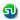Stumble Itdigg this